# Free online courseCalculus 1 by Leonard

4.62

(58)

## 44h56m of online video course

Quality content and selected for your learning.

## Exercises to train your knowledge

Many of the courses available have exercises to help with learning.

This course is free today and always will be, from start to finish you will pay nothing.

## Calculus

You can use our courses of Calculus in a lot of ways, like in physics, mathematics, economics and many other and here for sure is the best place to learnTeacher

##### Leonard
###### 6 Courses / 1743 students

This Channel is dedicated to quality mathematics education. It is absolutely FREE so Enjoy! Videos are organized in playlists and are course specific.

## Course content preview

There are no comments yet for this course.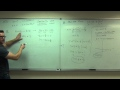Watch the 1st video of the course

## Course content0h48m

### Calculus 1 Lecture 0.1: Lines, Angle of Inclination, and the Distance Formula

Calculus 1 Lecture 0.1: Lines, Angle of Inclination, and the Distance Formula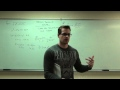1h37m

### Calculus 1 Lecture 0.2: Introduction to Functions.

Calculus 1 Lecture 0.2: Introduction to Functions.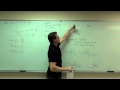1h20m

### Calculus 1 Lecture 0.3: Review of Trigonometry and Graphing Trigonometric Functions

Calculus 1 Lecture 0.3: Review of Trigonometry and Graphing Trigonometric Functions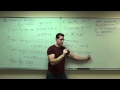0h15m

### Calculus 1 Lecture 0.4: Combining and Composition of Functions

Calculus 1 Lecture 0.4: Combining and Composition of Functions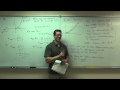1h27m

### Calculus 1 Lecture 1.1: An Introduction to Limits

Calculus 1 Lecture 1.1: An Introduction to Limits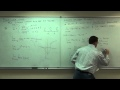3h00m

### Calculus 1 Lecture 1.2: Properties of Limits. Techniques of Limit Computation

Calculus 1 Lecture 1.2: Properties of Limits. Techniques of Limit Computation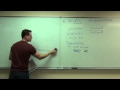1h26m

### Calculus 1 Lecture 1.4: Continuity of Functions

Calculus 1 Lecture 1.4: Continuity of Functions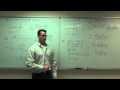1h50m

### Calculus 1 Lecture 1.5: Slope of a Curve, Velocity, and Rates of Change

Calculus 1 Lecture 1.5: Slope of a Curve, Velocity, and Rates of Change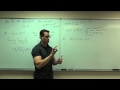1h16m

### Calculus 1 Lecture 2.1: Introduction to the Derivative of a Function

Calculus 1 Lecture 2.1: Introduction to the Derivative of a Function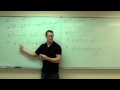1h12m

### Calculus 1 Lecture 2.2: Techniques of Differentiation (Finding Derivatives of Functions Easily)

Calculus 1 Lecture 2.2: Techniques of Differentiation (Finding Derivatives of Functions Easily)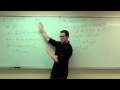1h02m

### Calculus 1 Lecture 2.3: The Product and Quotient Rules for Derivatives of Functions

Calculus 1 Lecture 2.3: The Product and Quotient Rules for Derivatives of Functions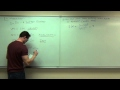0h40m

### Calculus 1 Lecture 2.4: Applications of the Derivative

Calculus 1 Lecture 2.4: Applications of the Derivative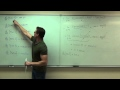0h48m

### Calculus 1 Lecture 2.5: Finding Derivatives of Trigonometric Functions

Calculus 1 Lecture 2.5: Finding Derivatives of Trigonometric Functions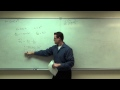1h34m

### Calculus 1 Lecture 2.6: Discussion of the Chain Rule for Derivatives of Functions

Calculus 1 Lecture 2.6: Discussion of the Chain Rule for Derivatives of Functions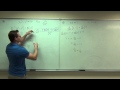1h08m

### Calculus 1 Lecture 2.7: Implicit Differentiation

Calculus 1 Lecture 2.7: Implicit Differentiation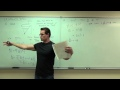0h53m

### Calculus 1 Lecture 2.8: Related Rates

Calculus 1 Lecture 2.8: Related Rates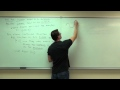1h34m

### Calculus 1 Lecture 3.1: Increasing/Decreasing and Concavity of Functions

Calculus 1 Lecture 3.1: Discussion of Increasing and Decreasing Intervals. Discussion of Concavity of functions.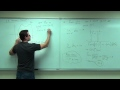0h06m

### Calculus 1 Lecture 3.2: A BRIEF Discussion of Rolle's Theorem and Mean-Value Theorem.

Calculus 1 Lecture 3.2: A BRIEF Discussion of Rolle's Theorem and Mean-Value Theorem.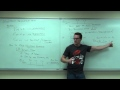0h26m

### Calculus 1 Lecture 3.3: The First Derivative Test for Increasing and Decreasing

Calculus 1 Lecture 3.3: The First Derivative Test for Increasing and Decreasing of Functions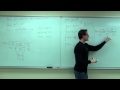0h36m

### Calculus 1 Lecture 3.4: The Second Derivative Test for Concavity of Functions

Calculus 1 Lecture 3.4: The Second Derivative Test for Concavity of Functions